# Stackelberg Plans¶

In addition to what’s in Anaconda, this lecture will need the following libraries:

In :
!pip install --upgrade quantecon


## Overview¶

This notebook formulates and computes a plan that a Stackelberg leader uses to manipulate forward-looking decisions of a Stackelberg follower that depend on continuation sequences of decisions made once and for all by the Stackelberg leader at time $0$.

To facilitate computation and interpretation, we formulate things in a context that allows us to apply dynamic programming for linear-quadratic models.

From the beginning, we carry along a linear-quadratic model of duopoly in which firms face adjustment costs that make them want to forecast actions of other firms that influence future prices.

In :
import numpy as np
import numpy.linalg as la
import quantecon as qe
from quantecon import LQ
import matplotlib.pyplot as plt
%matplotlib inline

/home/ubuntu/anaconda3/lib/python3.7/site-packages/numba/np/ufunc/parallel.py:355: NumbaWarning: The TBB threading layer requires TBB version 2019.5 or later i.e., TBB_INTERFACE_VERSION >= 11005. Found TBB_INTERFACE_VERSION = 11004. The TBB threading layer is disabled.
warnings.warn(problem)


## Duopoly¶

Time is discrete and is indexed by $t = 0, 1, \ldots$.

Two firms produce a single good whose demand is governed by the linear inverse demand curve

$$p_t = a_0 - a_1 (q_{1t}+ q_{2t} )$$

where $q_{it}$ is output of firm $i$ at time $t$ and $a_0$ and $a_1$ are both positive.

$q_{10}, q_{20}$ are given numbers that serve as initial conditions at time $0$.

By incurring a cost of change

$$\gamma v_{it}^2$$

where $\gamma > 0$, firm $i$ can change its output according to

$$q_{it+1} = q_{it} + v_{it}$$

Firm $i$’s profits at time $t$ equal

$$\pi_{it} = p_t q_{it} - \gamma v_{it}^2$$

Firm $i$ wants to maximize the present value of its profits

$$\sum_{t=0}^\infty \beta^t \pi_{it}$$

where $\beta \in (0,1)$ is a time discount factor.

Each firm $i=1,2$ chooses a sequence $\vec q_i \equiv \{q_{it+1}\}_{t=0}^\infty$ once and for all at time $0$.

We let firm 2 be a Stackelberg leader and firm 1 be a Stackelberg follower.

The leader firm 2 goes first and chooses $\{q_{2t+1}\}_{t=0}^\infty$ once and for all at time $0$.

Knowing that firm 2 has chosen $\{q_{2t+1}\}_{t=0}^\infty$, the follower firm 1 goes second and chooses $\{q_{1t+1}\}_{t=0}^\infty$ once and for all at time $0$.

In choosing $\vec q_2$, firm 2 takes into account that firm 1 will base its choice of $\vec q_1$ on firm 2’s choice of $\vec q_2$.

### Abstract Statement of the Leader’s and Follower’s Problems¶

We can express firm 1’s problem as

$$\max_{\vec q_1} \Pi_1(\vec q_1; \vec q_2)$$

where the appearance behind the semi-colon indicates that $\vec q_2$ is given.

Firm 1’s problem induces the best response mapping

$$\vec q_1 = B(\vec q_2)$$

(Here $B$ maps a sequence into a sequence)

$$\max_{\vec q_2} \Pi_2 (B(\vec q_2), \vec q_2)$$

whose maximizer is a sequence $\vec q_2$ that depends on the initial conditions $q_{10}, q_{20}$ and the parameters of the model $a_0, a_1, \gamma$.

This formulation captures key features of the model

• Both firms make once-and-for-all choices at time $0$.
• This is true even though both firms are choosing sequences of quantities that are indexed by time.
• The Stackelberg leader chooses first within time $0$, knowing that the Stackelberg follower will choose second within time $0$.

While our abstract formulation reveals the timing protocol and equilibrium concept well, it obscures details that must be addressed when we want to compute and interpret a Stackelberg plan and the follower’s best response to it.

To gain insights about these things, we study them in more detail.

### Firms’ Problems¶

Firm 1 acts as if firm 2’s sequence $\{q_{2t+1}\}_{t=0}^\infty$ is given and beyond its control.

Firm 2 knows that firm 1 chooses second and takes this into account in choosing $\{q_{2t+1}\}_{t=0}^\infty$.

In the spirit of working backward, we study firm 1’s problem first, taking $\{q_{2t+1}\}_{t=0}^\infty$ as given.

We can formulate firm 1’s optimum problem in terms of the Lagrangian

$$L=\sum_{t=0}^{\infty}\beta^{t}\{a_{0}q_{1t}-a_{1}q_{1t}^{2}-a_{1}q_{1t}q_{2t}-\gamma v_{1t}^{2}+\lambda_{t}[q_{1t}+v_{1t}-q_{1t+1}]\}$$

Firm 1 seeks a maximum with respect to $\{q_{1t+1}, v_{1t} \}_{t=0}^\infty$ and a minimum with respect to $\{ \lambda_t\}_{t=0}^\infty$.

We approach this problem using methods described in Ljungqvist and Sargent RMT5 chapter 2, appendix A and Macroeconomic Theory, 2nd edition, chapter IX.

First-order conditions for this problem are

\begin{aligned} \frac{\partial L}{\partial q_{1t}} & = a_0 - 2 a_1 q_{1t} - a_1 q_{2t} + \lambda_t - \beta^{-1} \lambda_{t-1} = 0 , \quad t \geq 1 \cr \frac{\partial L}{\partial v_{1t}} & = -2 \gamma v_{1t} + \lambda_t = 0 , \quad t \geq 0 \cr \end{aligned}

These first-order conditions and the constraint $q_{1t+1} = q_{1t} + v_{1t}$ can be rearranged to take the form

\begin{aligned} v_{1t} & = \beta v_{1t+1} + \frac{\beta a_0}{2 \gamma} - \frac{\beta a_1}{\gamma} q_{1t+1} - \frac{\beta a_1}{2 \gamma} q_{2t+1} \cr q_{t+1} & = q_{1t} + v_{1t} \end{aligned}

We can substitute the second equation into the first equation to obtain

$$(q_{1t+1} - q_{1t} ) = \beta (q_{1t+2} - q_{1t+1}) + c_0 - c_1 q_{1t+1} - c_2 q_{2t+1}$$

where $c_0 = \frac{\beta a_0}{2 \gamma}, c_1 = \frac{\beta a_1}{\gamma}, c_2 = \frac{\beta a_1}{2 \gamma}$.

This equation can in turn be rearranged to become the second-order difference equation

$$q_{1t} + (1+\beta + c_1) q_{1t+1} - \beta q_{1t+2} = c_0 - c_2 q_{2t+1} \tag{1}$$

Equation (1) is a second-order difference equation in the sequence $\vec q_1$ whose solution we want.

It satisfies two boundary conditions:

• an initial condition that $q_{1,0}$, which is given
• a terminal condition requiring that $\lim_{T \rightarrow + \infty} \beta^T q_{1t}^2 < + \infty$

Using the lag operators described in chapter IX of Macroeconomic Theory, Second edition (1987), difference equation (1) can be written as

$$\beta(1 - \frac{1+\beta + c_1}{\beta} L + \beta^{-1} L^2 ) q_{1t+2} = - c_0 + c_2 q_{2t+1}$$

The polynomial in the lag operator on the left side can be factored as

$$(1 - \frac{1+\beta + c_1}{\beta} L + \beta^{-1} L^2 ) = ( 1 - \delta_1 L ) (1 - \delta_2 L) \tag{2}$$

where $0 < \delta_1 < 1 < \frac{1}{\sqrt{\beta}} < \delta_2$.

Because $\delta_2 > \frac{1}{\sqrt{\beta}}$ the operator $(1 - \delta_2 L)$ contributes an unstable component if solved backwards but a stable component if solved forwards.

Mechanically, write

$$(1- \delta_2 L) = -\delta_{2} L (1 - \delta_2^{-1} L^{-1} )$$

and compute the following inverse operator

$$\left[-\delta_{2} L (1 - \delta_2^{-1} L^{-1} )\right]^{-1} = - \delta_2 (1 - {\delta_2}^{-1} )^{-1} L^{-1}$$

Operating on both sides of equation (2) with $\beta^{-1}$ times this inverse operator gives the follower’s decision rule for setting $q_{1t+1}$ in the feedback-feedforward form.

$$q_{1t+1} = \delta_1 q_{1t} - c_0 \delta_2^{-1} \beta^{-1} \frac{1}{1 -\delta_2^{-1}} + c_2 \delta_2^{-1} \beta^{-1} \sum_{j=0}^\infty \delta_2^j q_{2t+j+1} , \quad t \geq 0 \tag{3}$$

The problem of the Stackelberg leader firm 2 is to choose the sequence $\{q_{2t+1}\}_{t=0}^\infty$ to maximize its discounted profits

$$\sum_{t=0}^\infty \beta^t \{ (a_0 - a_1 (q_{1t} + q_{2t}) ) q_{2t} - \gamma (q_{2t+1} - q_{2t})^2 \}$$

subject to the sequence of constraints (3) for $t \geq 0$.

We can put a sequence $\{\theta_t\}_{t=0}^\infty$ of Lagrange multipliers on the sequence of equations (3) and formulate the following Lagrangian for the Stackelberg leader firm 2’s problem

\begin{aligned} \tilde L & = \sum_{t=0}^\infty \beta^t\{ (a_0 - a_1 (q_{1t} + q_{2t}) ) q_{2t} - \gamma (q_{2t+1} - q_{2t})^2 \} \cr & + \sum_{t=0}^\infty \beta^t \theta_t \{ \delta_1 q_{1t} - c_0 \delta_2^{-1} \beta^{-1} \frac{1}{1 -\delta_2^{-1}} + c_2 \delta_2^{-1} \beta^{-1} \sum_{j=0}^\infty \delta_2^{-j} q_{2t+j+1} - q_{1t+1} \} \end{aligned} \tag{4}

subject to initial conditions for $q_{1t}, q_{2t}$ at $t=0$.

Comments: We have formulated the Stackelberg problem in a space of sequences.

The max-min problem associated with Lagrangian (4) is unpleasant because the time $t$ component of firm $1$’s payoff function depends on the entire future of its choices of $\{q_{1t+j}\}_{j=0}^\infty$.

This renders a direct attack on the problem cumbersome.

Therefore, below, we will formulate the Stackelberg leader’s problem recursively.

We’ll put our little duopoly model into a broader class of models with the same conceptual structure.

## The Stackelberg Problem¶

We formulate a class of linear-quadratic Stackelberg leader-follower problems of which our duopoly model is an instance.

We use the optimal linear regulator (a.k.a. the linear-quadratic dynamic programming problem described in LQ Dynamic Programming problems) to represent a Stackelberg leader’s problem recursively.

Let $z_t$ be an $n_z \times 1$ vector of natural state variables.

Let $x_t$ be an $n_x \times 1$ vector of endogenous forward-looking variables that are physically free to jump at $t$.

In our duopoly example $x_t = v_{1t}$, the time $t$ decision of the Stackelberg follower.

Let $u_t$ be a vector of decisions chosen by the Stackelberg leader at $t$.

The $z_t$ vector is inherited physically from the past.

But $x_t$ is a decision made by the Stackelberg follower at time $t$ that is the follower’s best response to the choice of an entire sequence of decisions made by the Stackelberg leader at time $t=0$.

Let

$$y_t = \begin{bmatrix} z_t \\ x_t \end{bmatrix}$$

Represent the Stackelberg leader’s one-period loss function as

$$r(y, u) = y' R y + u' Q u$$

Subject to an initial condition for $z_0$, but not for $x_0$, the Stackelberg leader wants to maximize

$$-\sum_{t=0}^\infty \beta^t r(y_t, u_t) \tag{5}$$

The Stackelberg leader faces the model

$$\begin{bmatrix} I & 0 \\ G_{21} & G_{22} \end{bmatrix} \begin{bmatrix} z_{t+1} \\ x_{t+1} \end{bmatrix} = \begin{bmatrix} \hat A_{11} & \hat A_{12} \\ \hat A_{21} & \hat A_{22} \end{bmatrix} \begin{bmatrix} z_t \\ x_t \end{bmatrix} + \hat B u_t \tag{6}$$

We assume that the matrix $\begin{bmatrix} I & 0 \\ G_{21} & G_{22} \end{bmatrix}$ on the left side of equation (6) is invertible, so that we can multiply both sides by its inverse to obtain

$$\begin{bmatrix} z_{t+1} \\ x_{t+1} \end{bmatrix} = \begin{bmatrix} A_{11} & A_{12} \\ A_{21} & A_{22} \end{bmatrix} \begin{bmatrix} z_t \\ x_t \end{bmatrix} + B u_t \tag{7}$$

or

$$y_{t+1} = A y_t + B u_t \tag{8}$$

### Interpretation of the Second Block of Equations¶

The Stackelberg follower’s best response mapping is summarized by the second block of equations of (7).

In particular, these equations are the first-order conditions of the Stackelberg follower’s optimization problem (i.e., its Euler equations).

These Euler equations summarize the forward-looking aspect of the follower’s behavior and express how its time $t$ decision depends on the leader’s actions at times $s \geq t$.

When combined with a stability condition to be imposed below, the Euler equations summarize the follower’s best response to the sequence of actions by the leader.

The Stackelberg leader maximizes (5) by choosing sequences $\{u_t, x_t, z_{t+1}\}_{t=0}^{\infty}$ subject to (8) and an initial condition for $z_0$.

Note that we have an initial condition for $z_0$ but not for $x_0$.

$x_0$ is among the variables to be chosen at time $0$ by the Stackelberg leader.

The Stackelberg leader uses its understanding of the responses restricted by (8) to manipulate the follower’s decisions.

### More Mechanical Details¶

For any vector $a_t$, define $\vec a_t = [a_t, a_{t+1} \ldots ]$.

Define a feasible set of $(\vec y_1, \vec u_0)$ sequences

$$\Omega(y_0) = \left\{ (\vec y_1, \vec u_0) : y_{t+1} = A y_t + B u_t, \forall t \geq 0 \right\}$$

Please remember that the follower’s Euler equation is embedded in the system of dynamic equations $y_{t+1} = A y_t + B u_t$.

Note that in the definition of $\Omega(y_0)$, $y_0$ is taken as given.

Although it is taken as given in $\Omega(y_0)$, eventually, the $x_0$ component of $y_0$ will be chosen by the Stackelberg leader.

### Two Subproblems¶

Once again we use backward induction.

We express the Stackelberg problem in terms of two subproblems.

Subproblem 1 is solved by a continuation Stackelberg leader at each date $t \geq 0$.

Subproblem 2 is solved by the Stackelberg leader at $t=0$.

The two subproblems are designed

• to respect the protocol in which the follower chooses $\vec q_1$ after seeing $\vec q_2$ chosen by the leader
• to make the leader choose $\vec q_2$ while respecting that $\vec q_1$ will be the follower’s best response to $\vec q_2$
• to represent the leader’s problem recursively by artfully choosing the state variables confronting and the control variables available to the leader

#### Subproblem 1¶

$$v(y_0) = \max_{(\vec y_1, \vec u_0) \in \Omega(y_0)} - \sum_{t=0}^\infty \beta^t r(y_t, u_t)$$

#### Subproblem 2¶

$$w(z_0) = \max_{x_0} v(y_0)$$

Subproblem 1 takes the vector of forward-looking variables $x_0$ as given.

Subproblem 2 optimizes over $x_0$.

The value function $w(z_0)$ tells the value of the Stackelberg plan as a function of the vector of natural state variables at time $0$, $z_0$.

### Two Bellman Equations¶

We now describe Bellman equations for $v(y)$ and $w(z_0)$.

#### Subproblem 1¶

The value function $v(y)$ in subproblem 1 satisfies the Bellman equation

$$v(y) = \max_{u, y^*} \left\{ - r(y,u) + \beta v(y^*) \right\} \tag{9}$$

where the maximization is subject to

$$y^* = A y + B u$$

and $y^*$ denotes next period’s value.

Substituting $v(y) = - y'P y$ into Bellman equation (9) gives

$$-y' P y = {\rm max}_{ u, y^*} \left\{ - y' R y - u'Q u - \beta y^{* \prime} P y^* \right\}$$

which as in lecture linear regulator gives rise to the algebraic matrix Riccati equation

$$P = R + \beta A' P A - \beta^2 A' P B ( Q + \beta B' P B)^{-1} B' P A$$

and the optimal decision rule coefficient vector

$$F = \beta( Q + \beta B' P B)^{-1} B' P A$$

where the optimal decision rule is

$$u_t = - F y_t$$

#### Subproblem 2¶

We find an optimal $x_0$ by equating to zero the gradient of $v(y_0)$ with respect to $x_0$:

$$-2 P_{21} z_0 - 2 P_{22} x_0 =0,$$

which implies that

$$x_0 = - P_{22}^{-1} P_{21} z_0$$

## Stackelberg Plan¶

Now let’s map our duopoly model into the above setup.

We will formulate a state space system

$$y_t = \begin{bmatrix} z_t \cr x_t \end{bmatrix}$$

where in this instance $x_t = v_{1t}$, the time $t$ decision of the follower firm 1.

### Calculations to Prepare Duopoly Model¶

Now we’ll proceed to cast our duopoly model within the framework of the more general linear-quadratic structure described above.

That will allow us to compute a Stackelberg plan simply by enlisting a Riccati equation to solve a linear-quadratic dynamic program.

As emphasized above, firm 1 acts as if firm 2’s decisions $\{q_{2t+1}, v_{2t}\}_{t=0}^\infty$ are given and beyond its control.

### Firm 1’s Problem¶

We again formulate firm 1’s optimum problem in terms of the Lagrangian

$$L=\sum_{t=0}^{\infty}\beta^{t}\{a_{0}q_{1t}-a_{1}q_{1t}^{2}-a_{1}q_{1t}q_{2t}-\gamma v_{1t}^{2}+\lambda_{t}[q_{1t}+v_{1t}-q_{1t+1}]\}$$

Firm 1 seeks a maximum with respect to $\{q_{1t+1}, v_{1t} \}_{t=0}^\infty$ and a minimum with respect to $\{ \lambda_t\}_{t=0}^\infty$.

First-order conditions for this problem are

\begin{aligned} \frac{\partial L}{\partial q_{1t}} & = a_0 - 2 a_1 q_{1t} - a_1 q_{2t} + \lambda_t - \beta^{-1} \lambda_{t-1} = 0 , \quad t \geq 1 \cr \frac{\partial L}{\partial v_{1t}} & = -2 \gamma v_{1t} + \lambda_t = 0 , \quad t \geq 0 \cr \end{aligned}

These first-order order conditions and the constraint $q_{1t+1} = q_{1t} + v_{1t}$ can be rearranged to take the form

\begin{aligned} v_{1t} & = \beta v_{1t+1} + \frac{\beta a_0}{2 \gamma} - \frac{\beta a_1}{\gamma} q_{1t+1} - \frac{\beta a_1}{2 \gamma} q_{2t+1} \cr q_{t+1} & = q_{1t} + v_{1t} \end{aligned}

We use these two equations as components of the following linear system that confronts a Stackelberg continuation leader at time $t$

$$\begin{bmatrix} 1 & 0 & 0 & 0 \cr 0 & 1 & 0 & 0 \cr 0 & 0 & 1 & 0 \cr \frac{\beta a_0}{2 \gamma} & - \frac{\beta a_1}{2 \gamma} & -\frac{\beta a_1}{\gamma} & \beta \end{bmatrix} \begin{bmatrix} 1 \cr q_{2t+1} \cr q_{1t+1} \cr v_{1t+1} \end{bmatrix} = \begin{bmatrix} 1 & 0 & 0 & 0 \cr 0 & 1 & 0 & 0 \cr 0 & 0 & 1 & 1 \cr 0 & 0 & 0 & 1 \end{bmatrix} \begin{bmatrix} 1 \cr q_{2t} \cr q_{1t} \cr v_{1t} \end{bmatrix} + \begin{bmatrix} 0 \cr 1 \cr 0 \cr 0 \end{bmatrix} v_{2t}$$

Time $t$ revenues of firm 2 are $\pi_{2t} = a_0 q_{2t} - a_1 q_{2t}^2 - a_1 q_{1t} q_{2t}$ which evidently equal

$$z_t' R_1 z_t \equiv \begin{bmatrix} 1 \cr q_{2t} \cr q_{1t} \end{bmatrix}' \begin{bmatrix} 0 & \frac{a_0}{2}& 0 \cr \frac{a_0}{2} & -a_1 & -\frac{a_1}{2}\cr 0 & -\frac{a_1}{2} & 0 \end{bmatrix} \begin{bmatrix} 1 \cr q_{2t} \cr q_{1t} \end{bmatrix}$$

If we set $Q = \gamma$, then firm 2’s period $t$ profits can then be written

$$y_t' R y_t - Q v_{2t}^2$$

where

$$y_t = \begin{bmatrix} z_t \cr x_t \end{bmatrix}$$

with $x_t = v_{1t}$ and

$$R = \begin{bmatrix} R_1 & 0 \cr 0 & 0 \end{bmatrix}$$

We’ll report results of implementing this code soon.

But first, we want to represent the Stackelberg leader’s optimal choices recursively.

It is important to do this for several reasons:

• properly to interpret a representation of the Stackelberg leader’s choice as a sequence of history-dependent functions
• to formulate a recursive version of the follower’s choice problem

First, let’s get a recursive representation of the Stackelberg leader’s choice of $\vec q_2$ for our duopoly model.

## Recursive Representation of Stackelberg Plan¶

In order to attain an appropriate representation of the Stackelberg leader’s history-dependent plan, we will employ what amounts to a version of the Big K, little k device often used in macroeconomics by distinguishing $z_t$, which depends partly on decisions $x_t$ of the followers, from another vector $\check z_t$, which does not.

We will use $\check z_t$ and its history $\check z^t = [\check z_t, \check z_{t-1}, \ldots, \check z_0]$ to describe the sequence of the Stackelberg leader’s decisions that the Stackelberg follower takes as given.

Thus, we let $\check y_t' = \begin{bmatrix}\check z_t' & \check x_t'\end{bmatrix}$ with initial condition $\check z_0 = z_0$ given.

That we distinguish $\check z_t$ from $z_t$ is part and parcel of the Big K, little k device in this instance.

We have demonstrated that a Stackelberg plan for $\{u_t\}_{t=0}^\infty$ has a recursive representation

\begin{aligned} \check x_0 & = - P_{22}^{-1} P_{21} z_0 \cr u_t & = - F \check y_t, \quad t \geq 0 \cr \check y_{t+1} & = (A - BF) \check y_t, \quad t \geq 0 \end{aligned}

From this representation, we can deduce the sequence of functions $\sigma = \{\sigma_t(\check z^t)\}_{t=0}^\infty$ that comprise a Stackelberg plan.

For convenience, let $\check A \equiv A - BF$ and partition $\check A$ conformably to the partition $y_t = \begin{bmatrix}\check z_t \cr \check x_t \end{bmatrix}$ as

$$\begin{bmatrix}\check A_{11} & \check A_{12} \cr \check A_{21} & \check A_{22} \end{bmatrix}$$

Let $H^0_0 \equiv - P_{22}^{-1} P_{21}$ so that $\check x_0 = H^0_0 \check z_0$.

Then iterations on $\check y_{t+1} = \check A \check y_t$ starting from initial condition $\check y_0 = \begin{bmatrix}\check z_0 \cr H^0_0 \check z_0\end{bmatrix}$ imply that for $t \geq 1$

$$x_t = \sum_{j=1}^t H_j^t \check z_{t-j}$$

where

\begin{aligned} H^t_1 & = \check A_{21} \cr H^t_2 & = \check A_{22} \check A_{21} \cr \ \ \vdots \ \ & \ \ \quad \vdots \cr H^t_{t-1} & = \check A_{22}^{t-2} \check A_{21} \cr H^t_t & = \check A_{22}^{t-1}(\check A_{21} + \check A_{22} H^0_0 ) \end{aligned}

An optimal decision rule for the Stackelberg’s choice of $u_t$ is

$$u_t = - F \check y_t \equiv - \begin{bmatrix} F_z & F_x \cr \end{bmatrix} \begin{bmatrix}\check z_t \cr x_t \cr \end{bmatrix}$$

or

$$u_t = - F_z \check z_t - F_x \sum_{j=1}^t H^t_j z_{t-j} = \sigma_t(\check z^t) \tag{10}$$

Representation (10) confirms that whenever $F_x \neq 0$, the typical situation, the time $t$ component $\sigma_t$ of a Stackelberg plan is history-dependent, meaning that the Stackelberg leader’s choice $u_t$ depends not just on $\check z_t$ but on components of $\check z^{t-1}$.

After all, at the end of the day, it will turn out that because we set $\check z_0 = z_0$, it will be true that $z_t = \check z_t$ for all $t \geq 0$.

Then why did we distinguish $\check z_t$ from $z_t$?

The answer is that if we want to present to the Stackelberg follower a history-dependent representation of the Stackelberg leader’s sequence $\vec q_2$, we must use representation (10) cast in terms of the history $\check z^t$ and not a corresponding representation cast in terms of $z^t$.

### Dynamic Programming and Time Consistency of follower’s Problem¶

Given the sequence $\vec q_2$ chosen by the Stackelberg leader in our duopoly model, it turns out that the Stackelberg follower’s problem is recursive in the natural state variables that confront a follower at any time $t \geq 0$.

This means that the follower’s plan is time consistent.

To verify these claims, we’ll formulate a recursive version of a follower’s problem that builds on our recursive representation of the Stackelberg leader’s plan and our use of the Big K, little k idea.

### Recursive Formulation of a Follower’s Problem¶

We now use what amounts to another “Big $K$, little $k$” trick (see rational expectations equilibrium) to formulate a recursive version of a follower’s problem cast in terms of an ordinary Bellman equation.

Firm 1, the follower, faces $\{q_{2t}\}_{t=0}^\infty$ as a given quantity sequence chosen by the leader and believes that its output price at $t$ satisfies

$$p_t = a_0 - a_1 ( q_{1t} + q_{2t}) , \quad t \geq 0$$

Our challenge is to represent $\{q_{2t}\}_{t=0}^\infty$ as a given sequence.

To do so, recall that under the Stackelberg plan, firm 2 sets output according to the $q_{2t}$ component of

$$y_{t+1} = \begin{bmatrix} 1 \cr q_{2t} \cr q_{1t} \cr x_t \end{bmatrix}$$

which is governed by

$$y_{t+1} = (A - BF) y_t$$

To obtain a recursive representation of a $\{q_{2t}\}$ sequence that is exogenous to firm 1, we define a state $\tilde y_t$

$$\tilde y_t = \begin{bmatrix} 1 \cr q_{2t} \cr \tilde q_{1t} \cr \tilde x_t \end{bmatrix}$$

that evolves according to

$$\tilde y_{t+1} = (A - BF) \tilde y_t$$

subject to the initial condition $\tilde q_{10} = q_{10}$ and $\tilde x_0 = x_0$ where $x_0 = - P_{22}^{-1} P_{21}$ as stated above.

Firm 1’s state vector is

$$X_t = \begin{bmatrix} \tilde y_t \cr q_{1t} \end{bmatrix}$$

It follows that the follower firm 1 faces law of motion

$$\begin{bmatrix} \tilde y_{t+1} \\ q_{1t+1} \end{bmatrix} = \begin{bmatrix} A - BF & 0 \\ 0 & 1 \end{bmatrix} \begin{bmatrix} \tilde y_{t} \\ q_{1t} \end{bmatrix} + \begin{bmatrix} 0 \cr 1 \end{bmatrix} x_t \tag{11}$$

This specification assures that from the point of the view of a firm 1, $q_{2t}$ is an exogenous process.

Here

• $\tilde q_{1t}, \tilde x_t$ play the role of Big K
• $q_{1t}, x_t$ play the role of little k

The time $t$ component of firm 1’s objective is

$$\tilde X_t' \tilde R x_t - x_t^2 \tilde Q = \begin{bmatrix} 1 \cr q_{2t} \cr \tilde q_{1t} \cr \tilde x_t \cr q_{1t} \end{bmatrix}' \begin{bmatrix} 0 & 0 & 0 & 0 & \frac{a_0}{2} \cr 0 & 0 & 0 & 0 & - \frac{a_1}{2} \cr 0 & 0 & 0 & 0 & 0 \cr 0 & 0 & 0 & 0 & 0 \cr \frac{a_0}{2} & -\frac{a_1}{2} & 0 & 0 & - a_1 \end{bmatrix} \begin{bmatrix} 1 \cr q_{2t} \cr \tilde q_{1t} \cr \tilde x_t \cr q_{1t} \end{bmatrix} - \gamma x_t^2$$

Firm 1’s optimal decision rule is

$$x_t = - \tilde F X_t$$

and it’s state evolves according to

$$\tilde X_{t+1} = (\tilde A - \tilde B \tilde F) X_t$$

under its optimal decision rule.

Later we shall compute $\tilde F$ and verify that when we set

$$X_0 = \begin{bmatrix} 1 \cr q_{20} \cr q_{10} \cr x_0 \cr q_{10} \end{bmatrix}$$

we recover

$$x_0 = - \tilde F \tilde X_0$$

which will verify that we have properly set up a recursive representation of the follower’s problem facing the Stackelberg leader’s $\vec q_2$.

### Time Consistency of Follower’s Plan¶

Since the follower can solve its problem using dynamic programming its problem is recursive in what for it are the natural state variables, namely

$$\begin{bmatrix} 1 \cr q_{2t} \cr \tilde q_{10} \cr \tilde x_0 \end{bmatrix}$$

It follows that the follower’s plan is time consistent.

## Computing the Stackelberg Plan¶

Here is our code to compute a Stackelberg plan via a linear-quadratic dynamic program as outlined above

In :
# Parameters
a0 = 10
a1 = 2
β = 0.96
γ = 120
n = 300
tol0 = 1e-8
tol1 = 1e-16
tol2 = 1e-2

βs = np.ones(n)
βs[1:] = β
βs = βs.cumprod()

In :
# In LQ form
Alhs = np.eye(4)

# Euler equation coefficients
Alhs[3, :] = β * a0 / (2 * γ), -β * a1 / (2 * γ), -β * a1 / γ, β

Arhs = np.eye(4)
Arhs[2, 3] = 1

Alhsinv = la.inv(Alhs)

A = Alhsinv @ Arhs

B = Alhsinv @ np.array([[0, 1, 0, 0]]).T

R = np.array([[0,      -a0 / 2,      0, 0],
[-a0 / 2,     a1, a1 / 2, 0],
[0,       a1 / 2,      0, 0],
[0,            0,      0, 0]])

Q = np.array([[γ]])

# Solve using QE's LQ class
# LQ solves minimization problems which is why the sign of R and Q was changed
lq = LQ(Q, R, A, B, beta=β)
P, F, d = lq.stationary_values(method='doubling')

P22 = P[3:, 3:]
P21 = P[3:, :3]
P22inv = la.inv(P22)
H_0_0 = -P22inv @ P21

# Simulate forward

z0 = np.array([[1, 1, 1]]).T
x0 = H_0_0 @ z0
y0 = np.vstack((z0, x0))

yt, ut = lq.compute_sequence(y0, ts_length=n)[:2]

π_matrix = (R + F. T @ Q @ F)

for t in range(n):
π_leader[t] = -(yt[:, t].T @ π_matrix @ yt[:, t])

# Display policies
print(f"F = {F}")

Computed policy for Stackelberg leader

F = [[-1.58004454  0.29461313  0.67480938  6.53970594]]


### Implied Time Series for Price and Quantities¶

The following code plots the price and quantities

In :
q_leader = yt[1, :-1]
q_follower = yt[2, :-1]
q = q_leader + q_follower       # Total output, Stackelberg
p = a0 - a1 * q                 # Price, Stackelberg

fig, ax = plt.subplots(figsize=(9, 5.8))
ax.plot(range(n), q_follower, 'r-', lw=2, label='follower output')
ax.plot(range(n), p, 'g-', lw=2, label='price')
ax.set_title('Output and prices, Stackelberg duopoly')
ax.legend(frameon=False)
ax.set_xlabel('t')
plt.show()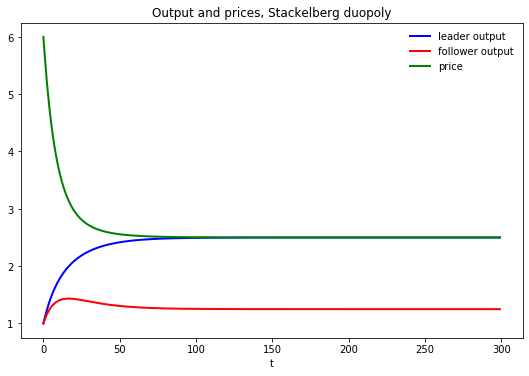We’ll compute the present value earned by the Stackelberg leader.

We’ll compute it two ways (they give identical answers – just a check on coding and thinking)

In :
v_leader_forward = np.sum(βs * π_leader)
v_leader_direct = -yt[:, 0].T @ P @ yt[:, 0]

# Display values
print("Computed values for the Stackelberg leader at t=0:\n")

Computed values for the Stackelberg leader at t=0:


In :
# Manually checks whether P is approximately a fixed point
P_next = (R + F.T @ Q @ F + β * (A - B @ F).T @ P @ (A - B @ F))
(P - P_next < tol0).all()

Out:
True
In :
# Manually checks whether two different ways of computing the
# value function give approximately the same answer
v_expanded = -((y0.T @ R @ y0 + ut[:, 0].T @ Q @ ut[:, 0] +
β * (y0.T @ (A - B @ F).T @ P @ (A - B @ F) @ y0)))
(v_leader_direct - v_expanded < tol0)[0, 0]

Out:
True

## Exhibiting Time Inconsistency of Stackelberg Plan¶

In the code below we compare two values

• the continuation value $- y_t P y_t$ earned by a continuation Stackelberg leader who inherits state $y_t$ at $t$
• the value of a reborn Stackelberg leader who inherits state $z_t$ at $t$ and sets $x_t = - P_{22}^{-1} P_{21}$

The difference between these two values is a tell-tale sign of the time inconsistency of the Stackelberg plan

In :
# Compute value function over time with a reset at time t

yt_reset = yt.copy()
yt_reset[-1, :] = (H_0_0 @ yt[:3, :])

for t in range(n):
vt_leader[t] = -yt[:, t].T @ P @ yt[:, t]
vt_reset_leader[t] = -yt_reset[:, t].T @ P @ yt_reset[:, t]

In :
fig, axes = plt.subplots(3, 1, figsize=(10, 7))

axes.plot(range(n+1), (- F @ yt).flatten(), 'bo',
axes.plot(range(n+1), (- F @ yt_reset).flatten(), 'ro',
axes.set(title=r'Leader control variable $u_{t}$', xlabel='t')
axes.legend()

axes.plot(range(n+1), yt[3, :], 'bo', ms=2)
axes.plot(range(n+1), yt_reset[3, :], 'ro', ms=2)
axes.set(title=r'Follower control variable $x_{t}$', xlabel='t')

axes.set(title=r'Leader value function $v(y_{t})$', xlabel='t')

plt.tight_layout()
plt.show()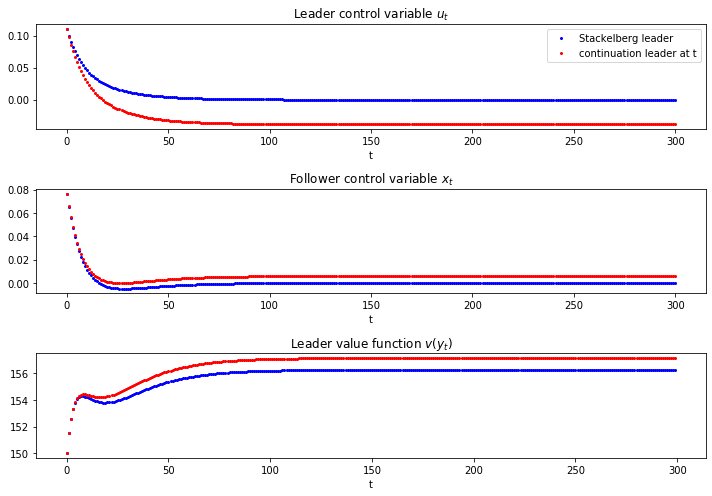## Recursive Formulation of the Follower’s Problem¶

We now formulate and compute the recursive version of the follower’s problem.

We check that the recursive Big $K$ , little $k$ formulation of the follower’s problem produces the same output path $\vec q_1$ that we computed when we solved the Stackelberg problem

In :
A_tilde = np.eye(5)
A_tilde[:4, :4] = A - B @ F

R_tilde = np.array([[0,            0, 0,    0, -a0 / 2],
[0,            0, 0,    0,  a1 / 2],
[0,            0, 0,    0,       0],
[0,            0, 0,    0,       0],
[-a0 / 2, a1 / 2, 0,    0,      a1]])

Q_tilde = Q
B_tilde = np.array([[0, 0, 0, 0, 1]]).T

lq_tilde = LQ(Q_tilde, R_tilde, A_tilde, B_tilde, beta=β)
P_tilde, F_tilde, d_tilde = lq_tilde.stationary_values(method='doubling')

y0_tilde = np.vstack((y0, y0))
yt_tilde = lq_tilde.compute_sequence(y0_tilde, ts_length=n)

In :
# Checks that the recursive formulation of the follower's problem gives
# the same solution as the original Stackelberg problem
fig, ax = plt.subplots()
ax.plot(yt_tilde, 'r', label="q_tilde")
ax.plot(yt_tilde, 'b', label="q")
ax.legend()
plt.show()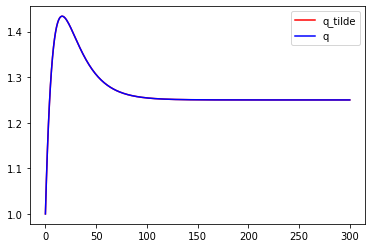Note: Variables with _tilde are obtained from solving the follower’s problem – those without are from the Stackelberg problem

In :
# Maximum absolute difference in quantities over time between
# the first and second solution methods
np.max(np.abs(yt_tilde - yt_tilde))

Out:
6.661338147750939e-16
In :
# x0 == x0_tilde
yt[:, 0][-1] - (yt_tilde[:, 1] - yt_tilde[:, 0])[-1] < tol0

Out:
True

### Explanation of Alignment¶

If we inspect the coefficients in the decision rule $- \tilde F$, we can spot the reason that the follower chooses to set $x_t = \tilde x_t$ when it sets $x_t = - \tilde F X_t$ in the recursive formulation of the follower problem.

Can you spot what features of $\tilde F$ imply this?

Hint: remember the components of $X_t$

In :
# Policy function in the follower's problem
F_tilde.round(4)

Out:
array([[-0.    ,  0.    , -0.1032, -1.    ,  0.1032]])
In :
# Value function in the Stackelberg problem
P

Out:
array([[  963.54083615,  -194.60534465,  -511.62197962, -5258.22585724],
[ -194.60534465,    37.3535753 ,    81.97712513,   784.76471234],
[ -511.62197962,    81.97712513,   247.34333344,  2517.05126111],
[-5258.22585724,   784.76471234,  2517.05126111, 25556.16504097]])
In :
# Value function in the follower's problem
P_tilde

Out:
array([[-1.81991134e+01,  2.58003020e+00,  1.56048755e+01,
1.51229815e+02, -5.00000000e+00],
[ 2.58003020e+00, -9.69465925e-01, -5.26007958e+00,
-5.09764310e+01,  1.00000000e+00],
[ 1.56048755e+01, -5.26007958e+00, -3.22759027e+01,
-3.12791908e+02, -1.23823802e+01],
[ 1.51229815e+02, -5.09764310e+01, -3.12791908e+02,
-3.03132584e+03, -1.20000000e+02],
[-5.00000000e+00,  1.00000000e+00, -1.23823802e+01,
-1.20000000e+02,  1.43823802e+01]])
In :
# Manually check that P is an approximate fixed point
(P  - ((R + F.T @ Q @ F) + β * (A - B @ F).T @ P @ (A - B @ F)) < tol0).all()

Out:
True
In :
# Compute P_guess using F_tilde_star
F_tilde_star = -np.array([[0, 0, 0, 1, 0]])
P_guess = np.zeros((5, 5))

for i in range(1000):
P_guess = ((R_tilde + F_tilde_star.T @ Q @ F_tilde_star) +
β * (A_tilde - B_tilde @ F_tilde_star).T @ P_guess
@ (A_tilde - B_tilde @ F_tilde_star))

In :
# Value function in the follower's problem
-(y0_tilde.T @ P_tilde @ y0_tilde)[0, 0]

Out:
112.65590740578058
In :
# Value function with P_guess
-(y0_tilde.T @ P_guess @ y0_tilde)[0, 0]

Out:
112.6559074057807
In :
# Compute policy using policy iteration algorithm
F_iter = (β * la.inv(Q + β * B_tilde.T @ P_guess @ B_tilde)
@ B_tilde.T @ P_guess @ A_tilde)

for i in range(100):
# Compute P_iter
P_iter = np.zeros((5, 5))
for j in range(1000):
P_iter = ((R_tilde + F_iter.T @ Q @ F_iter) + β
* (A_tilde - B_tilde @ F_iter).T @ P_iter
@ (A_tilde - B_tilde @ F_iter))

# Update F_iter
F_iter = (β * la.inv(Q + β * B_tilde.T @ P_iter @ B_tilde)
@ B_tilde.T @ P_iter @ A_tilde)

dist_vec = (P_iter - ((R_tilde + F_iter.T @ Q @ F_iter)
+ β * (A_tilde - B_tilde @ F_iter).T @ P_iter
@ (A_tilde - B_tilde @ F_iter)))

if np.max(np.abs(dist_vec)) < 1e-8:
dist_vec2 = (F_iter - (β * la.inv(Q + β * B_tilde.T @ P_iter @ B_tilde)
@ B_tilde.T @ P_iter @ A_tilde))

if np.max(np.abs(dist_vec2)) < 1e-8:
F_iter
else:
print("The policy didn't converge: try increasing the number of \
outer loop iterations")
else:
print("P_iter didn't converge: try increasing the number of inner \
loop iterations")

In :
# Simulate the system using F_tilde_star and check that it gives the
# same result as the original solution

yt_tilde_star = np.zeros((n, 5))
yt_tilde_star[0, :] = y0_tilde.flatten()

for t in range(n-1):
yt_tilde_star[t+1, :] = (A_tilde - B_tilde @ F_tilde_star) \
@ yt_tilde_star[t, :]

fig, ax = plt.subplots()
ax.plot(yt_tilde_star[:, 4], 'r', label="q_tilde")
ax.plot(yt_tilde, 'b', label="q")
ax.legend()
plt.show()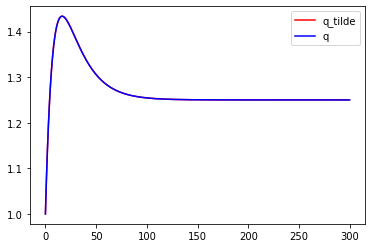In :
# Maximum absolute difference
np.max(np.abs(yt_tilde_star[:, 4] - yt_tilde[2, :-1]))

Out:
0.0

## Markov Perfect Equilibrium¶

The state vector is

$$z_t = \begin{bmatrix} 1 \cr q_{2t} \cr q_{1t} \end{bmatrix}$$

and the state transition dynamics are

$$z_{t+1} = A z_t + B_1 v_{1t} + B_2 v_{2t}$$

where $A$ is a $3 \times 3$ identity matrix and

$$B_1 = \begin{bmatrix} 0 \cr 0 \cr 1 \end{bmatrix} , \quad B_2 = \begin{bmatrix} 0 \cr 1 \cr 0 \end{bmatrix}$$

The Markov perfect decision rules are

$$v_{1t} = - F_1 z_t , \quad v_{2t} = - F_2 z_t$$

and in the Markov perfect equilibrium, the state evolves according to

$$z_{t+1} = (A - B_1 F_1 - B_2 F_2) z_t$$
In :
# In LQ form
A = np.eye(3)
B1 = np.array([, , ])
B2 = np.array([, , ])

R1 = np.array([[0,            0, -a0 / 2],
[0,            0,  a1 / 2],
[-a0 / 2, a1 / 2,      a1]])

R2 = np.array([[0,       -a0 / 2,      0],
[-a0 / 2,      a1, a1 / 2],
[0,        a1 / 2,      0]])

Q1 = Q2 = γ
S1 = S2 = W1 = W2 = M1 = M2 = 0.0

# Solve using QE's nnash function
F1, F2, P1, P2 = qe.nnash(A, B1, B2, R1, R2, Q1,
Q2, S1, S2, W1, W2, M1,
M2, beta=β, tol=tol1)

# Simulate forward
AF = A - B1 @ F1 - B2 @ F2
z = np.empty((3, n))
z[:, 0] = 1, 1, 1
for t in range(n-1):
z[:, t+1] = AF @ z[:, t]

# Display policies
print("Computed policies for firm 1 and firm 2:\n")
print(f"F1 = {F1}")
print(f"F2 = {F2}")

Computed policies for firm 1 and firm 2:

F1 = [[-0.22701363  0.03129874  0.09447113]]
F2 = [[-0.22701363  0.09447113  0.03129874]]

In :
q1 = z[1, :]
q2 = z[2, :]
q = q1 + q2       # Total output, MPE
p = a0 - a1 * q   # Price, MPE

fig, ax = plt.subplots(figsize=(9, 5.8))
ax.plot(range(n), q, 'b-', lw=2, label='total output')
ax.plot(range(n), p, 'g-', lw=2, label='price')
ax.set_title('Output and prices, duopoly MPE')
ax.legend(frameon=False)
ax.set_xlabel('t')
plt.show()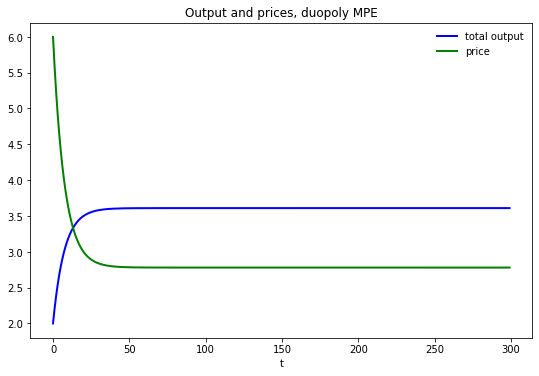In :
# Computes the maximum difference between the two quantities of the two firms
np.max(np.abs(q1 - q2))

Out:
6.8833827526759706e-15
In :
# Compute values
u1 = (- F1 @ z).flatten()
u2 = (- F2 @ z).flatten()

π_1 = p * q1 - γ * (u1) ** 2
π_2 = p * q2 - γ * (u2) ** 2

v1_forward = np.sum(βs * π_1)
v2_forward = np.sum(βs * π_2)

v1_direct = (- z[:, 0].T @ P1 @ z[:, 0])
v2_direct = (- z[:, 0].T @ P2 @ z[:, 0])

# Display values
print("Computed values for firm 1 and firm 2:\n")
print(f"v1(forward sim) = {v1_forward:.4f}; v1 (direct) = {v1_direct:.4f}")
print(f"v2 (forward sim) = {v2_forward:.4f}; v2 (direct) = {v2_direct:.4f}")

Computed values for firm 1 and firm 2:

v1(forward sim) = 133.3303; v1 (direct) = 133.3296
v2 (forward sim) = 133.3303; v2 (direct) = 133.3296

In :
# Sanity check
Λ1 = A - B2 @ F2
lq1 = qe.LQ(Q1, R1, Λ1, B1, beta=β)
P1_ih, F1_ih, d = lq1.stationary_values()

v2_direct_alt = - z[:, 0].T @ lq1.P @ z[:, 0] + lq1.d

(np.abs(v2_direct - v2_direct_alt) < tol2).all()

Out:
True

## MPE vs. Stackelberg¶

In :
vt_MPE = np.zeros(n)
vt_follower = np.zeros(n)

for t in range(n):
vt_MPE[t] = -z[:, t].T @ P1 @ z[:, t]
vt_follower[t] = -yt_tilde[:, t].T @ P_tilde @ yt_tilde[:, t]

fig, ax = plt.subplots()
ax.plot(vt_MPE, 'b', label='MPE')
ax.plot(vt_follower, 'g', label='Stackelberg follower')
ax.set_title(r'MPE vs. Stackelberg Value Function')
ax.set_xlabel('t')
ax.legend(loc=(1.05, 0))
plt.show()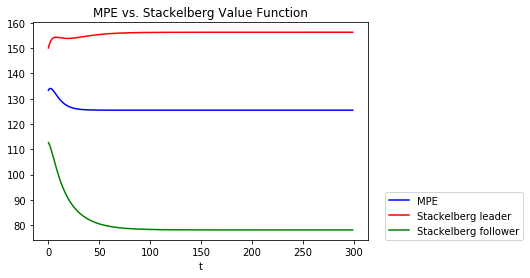In :
# Display values
print("Computed values:\n")
print(f"vt_follower(y0) = {vt_follower:.4f}")
print(f"vt_MPE(y0) = {vt_MPE:.4f}")

Computed values:


# Compute the difference in total value between the Stackelberg and the MPE

-3.970942562087714